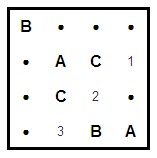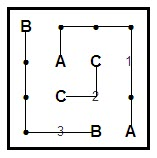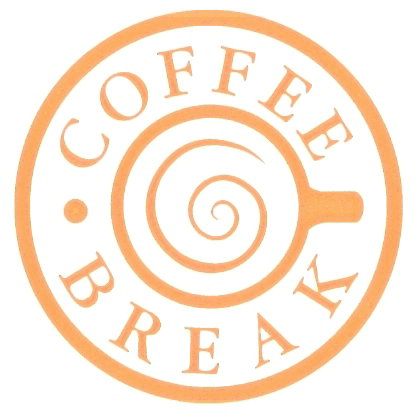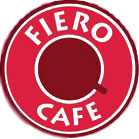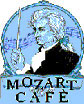### PUZZLE... PUZZLE... PUZZLE...

Games Editor: Hale Nur Kazaçeşme (MATH/V)

The objective is to fill in the grid with the digits 1 through 6 so that:

Each row and column contains exactly one of each digit; and Each bold-outlined group of cells is a cage containing digits that achieve the specified result using the specified mathematical operation: addition (+), subtraction (-), multiplication (×), and division (÷).

In this special case, these twin KenKen puzzles have the same solution.

When you finish, submit the contents of the fifth row to puzzle@bilkent.edu.tr

Send in your e-mail with the right answer and win! Prizes will be: dessert and coffee from Mozart Cafe (one each for three winners); coffee from Coffee Break (two each for two winners); hot chocolate from Cafe Fiero (one each for five winners); and chocolates from Bind Chocolate (two winners).

ALPHABET CONNECTION
Connect each pair of matching letters with a continuous line. The lines cannot cross each other.
In your answer, state which number is on which path. The solution for the example would be: 1A 2C 3B.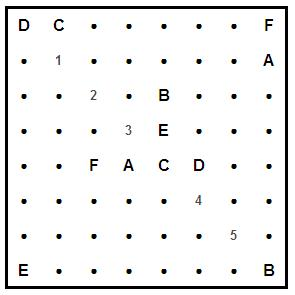Example